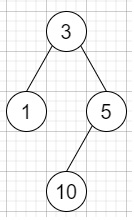# Kill Process in C++

Suppose we have n processes, here each process has a unique id called PID or process id and its PPID (parent process id) is also there.

Each process only has one parent process, but may have one or more child processes.

This is just like a tree structure. Only one process has the PPID = 0, which means this process has no parent process. All the PIDs will be unique positive integers.

We will use two list of integers to represent a list of processes, where the first list contains PID for each process and the second list contains the corresponding PPID. So, if we have two lists, and a PID representing a process we want to kill, then we have to find a list of PIDs of processes that will be killed in the end. And we should assume that when a process is killed, all its children processes will be killed.

So, if the input is like pid = [1, 3, 10, 5] ppid = [3, 0, 5, 3] kill = 5, then the output will be [5,10],Kill 5 will also kill 10.

To solve this, we will follow these steps −

• Define one map child

• n := size of pid

• Define an array ret

• for initialize i := 0, when i < n, update (increase i by 1), do −

• u := ppid[i]

• v := pid[i]

• insert v at the end of child[u]

• Define one queue q

• insert kill into q

• while (not q is empty), do −

• curr := first element of q

• delete element from q

• insert curr at the end of ret

• for initialize i := 0, when i < size of child[curr], update (increase i by 1), do −

• insert child[curr, i] into q

• return ret

## Example

Let us see the following implementation to get a better understanding −

Live Demo

#include <bits/stdc++.h>
using namespace std;
void print_vector(vector<auto> v){
cout << "[";
for(int i = 0; i<v.size(); i++){
cout << v[i] << ", ";
}
cout << "]"<<endl;
}
class Solution {
public:
vector<int> killProcess(vector<int>& pid, vector<int>& ppid, int kill) {
map<int, vector<int> > child;
int n = pid.size();
vector<int> ret;
for (int i = 0; i < n; i++) {
int u = ppid[i];
int v = pid[i];
child[u].push_back(v);
}
queue<int> q;
q.push(kill);
while (!q.empty()) {
int curr = q.front();
q.pop();
ret.push_back(curr);
for (int i = 0; i < child[curr].size(); i++) {
q.push(child[curr][i]);
}
}
return ret;
}
};
main(){
Solution ob;
vector<int> v = {1,3,10,5}, v1 = {3,0,5,3};
print_vector(ob.killProcess(v, v1, 5));
}

## Input

{1,3,10,5},{3,0,5,3},5

## Output

[5, 10, ]

Updated on: 16-Nov-2020

1K+ Views